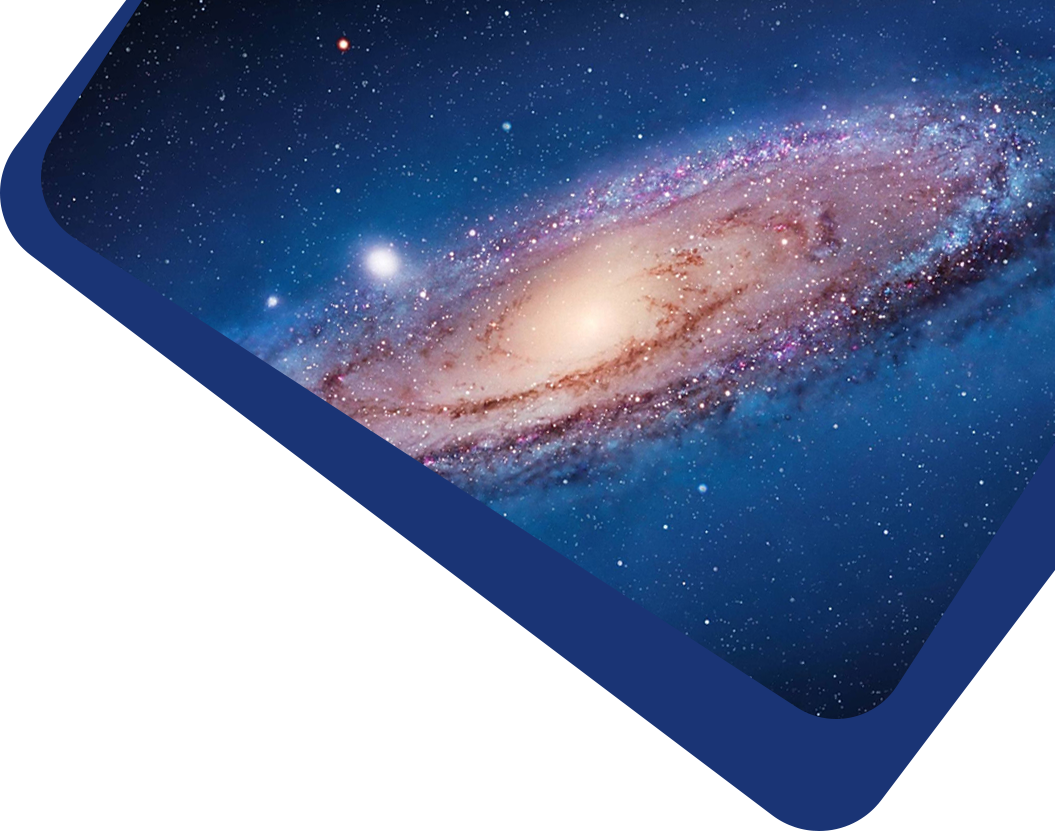Vol 23, No 3

## A Better Reconciliation of Hubble Tension in the Dark Energy Scalar Field

#### Le Fu, Li Chen, Maoyou Yang, Junmei Wang and Ming-Jian Zhang

Abstract

Hubble tension between the local measurement and global observation has been a key problem in cosmology. In this paper, we consider the quintessence scalar field, phantom field and quintom field as the dark energy to reconcile this problem. Different from most previous work, we start from the dimensionless equation of state (w) of dark energy, not a parameterization of potential. The combined analysis shows that observational data sets favor Hubble constant $$H_0=71.3^{+0.854}_{-0.917}\rm km\,s^{-1}\,Mpc^{-1}$$, which can reconcile Hubble tension within 1.20σ. We also perform a Bayes factor analysis using the MCEvidence code, and confirm that the phantom scalar field is still the most effective. To investigate the reason of Hubble tension, we analyze the density parameter. The comparison shows that the scalar fields provide a slightly larger Ωbh2 and smaller Ωch2 than the standard ΛCDM model. We finally analyze a possible reason of Hubble tension from the kinematic acceleration $$\ddot{a}$$. We find an interesting physical phenomenon. The acceleration $$\ddot{a}$$ in these scalar fields are similar as the ΛCDM model at about redshift z > 0.5. However, they increase and deviate from each other at low redshift, especially in the near future. Only the $$\ddot{a}$$ in phantom scalar field will decrease in the future.

Keywords

Full Text
Refbacks